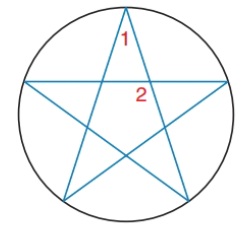Chapter 6.2, Problem 29E### Elementary Geometry for College St...

6th Edition
Daniel C. Alexander + 1 other
ISBN: 9781285195698

#### Solutions

Chapter
Section### Elementary Geometry for College St...

6th Edition
Daniel C. Alexander + 1 other
ISBN: 9781285195698
Textbook Problem
3 views

# For the five-pointed star (a regular pentagram) inscribed in the circle, find the measure of ∠ 1   and   ∠ 2 .To determine

To find:

The measure of 1and2.

Explanation

Given:

Calculation:

If 1 has the vertex on the circle:

mA=m1=mCD2=360°52=36°

If 2 has the vertex inside the circle.

m2=mAB+mCDE2=m

### Still sussing out bartleby?

Check out a sample textbook solution.

See a sample solution

#### The Solution to Your Study Problems

Bartleby provides explanations to thousands of textbook problems written by our experts, many with advanced degrees!

Get Started

#### Let g be the function defined by g(x) = x2 + 2x. Find g(a + h), g(a), g(a), a + g(a), and 1g(a).

Applied Calculus for the Managerial, Life, and Social Sciences: A Brief Approach

#### Find if , y = sin 2t.

Study Guide for Stewart's Multivariable Calculus, 8th

#### Let and g(x) = x + 3. Then (g ∘ h)(x) = ______.

Study Guide for Stewart's Single Variable Calculus: Early Transcendentals, 8th